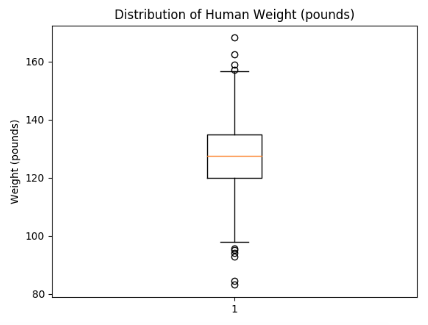top of page

# Summary

### Boxplots

There are multiple ways to visualize data other than a histogram, with which it can be difficult to see the median of the distribution. We can also visualize medians (and distributions) with another type of graph called a box plot. Let's look at our human weight data in box plot form.

This code should yield a box plot titled WeightBoxPlot.png that looks like this:Let's break this down. The red line in the middle, as you have likely guessed, is the median of the data. The upper blue line represents the 75th percentile of the data. The 75th percentile is the value at which 75% of the data points lie below it. So in this data, the 75th percentile is approximately 135, meaning that 75% of the data points are less than 135. The bottom blue line is the 25th percentile, which is the value at which 25% of the data points lie below it. So in this data, the 25th percentile is 120, meaning that 25% of the data points are less than 120.

The lines extending from either side of the box are called "whiskers" (box plots are also called box-and-whisker plots for this reason). They denote the range of the data; points outside this range are considered outliers and are plotted individually (the crosses on this graph).

So far, all the data we've looked at, even the data with a huge outlier, have been mostly symmetrical (except for the outlier itself) -- the shape of the histogram is relatively similar on both sides of the mean.

Sometimes data is very asymmetric, though. For example, let's look at the histogram generated with the code below.This generates a histogram titled AsymmetricHistogram.png, which should look something like this:

Lets calculate the exact mean and median of the above distribution to check our intuition.

This data is what we refer to as "skewed" -- one side of the histogram is significantly heavier than the other (in this case, the right side). This particular data would be called "right-skewed." The right skew is what causes the mean and median to differ from each other. Specifically, the middle value (the median) is close to 0, since clearly about half of the values are above it and half are below it. But the heavy right tail (the extra high values) pulls the mean upward so it is quite a bit higher than the median.

This example shows why we need different measures of our data ("summary statistics") -- for some distributions, the mean and median will be very similar, but in cases like this, they give very different information.

bottom of page